Normal view MARC view ISBD view

Springer series in computational mathematics. Vol 15. Mixed and hybrid finite element methods.

 No cover image available No cover image available No cover image available No cover image available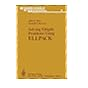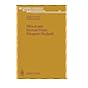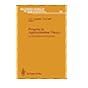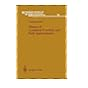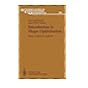519.62 Springer series in computational mathematics. Vol 3. Minimization methods for non-differentiable functions. 519.62 Springer series in computational mathematics. Vol 21. Numerical toolbox for verified computing 1. Basic numerical problems. 519.62 Springer series in computational mathematics. Vol 2. Solving elliptic problems using ELLPACK. 519.62 Springer series in computational mathematics. Vol 15. Mixed and hybrid finite element methods. 519.62 Springer series in computational mathematics. Vol 19. Progress in approximation theory. An international perspective. 519.62 Springer Series in Computational Mathematics Vol 12. History of continued fractions and Pade approximants. 519.62 Springer series in computational mathematics. Vol 16. Introduction to shape optimization : shape sensitivity analysis..